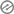# Primary 3 Mathematics First Term

Primary 3 Mathematics First Term

Weekly Lesson Plan

Week 1Writing Whole numbers from 1 to 999

Week 2Writing of whole numbers in wordsWeek 3

Comparison of Numbers by using greater than or less than

Week 4

Introduction of Fractions with shapes like triangle, square, rectangle, circle etcWeek 5

Addition of two digit numbers under tens and units without regroupingWeek 6

Comparison of Fractions by orderingWeek 7

Addition of three digits numbers without regroupingWeek 8Adding of two and three digit numbers with regroupingWeek 9

Addition of Fractions with the same denominatorsWeek 10

Subtraction of two digit numbers without regroupingWeek 11Subtraction of Numbers with 2 or 3 digit numbers with regrouping

Week 12Revision and Examination